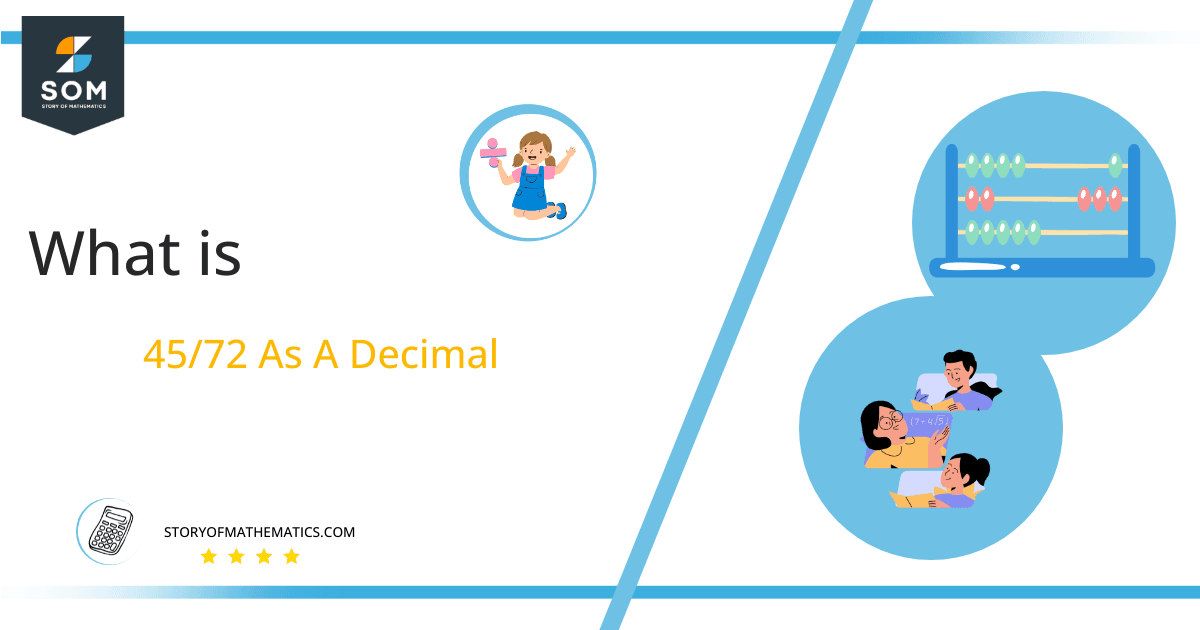# What Is 45/72 as a Decimal + Solution With Free Steps

The fraction 45/72 as a decimal is equal to 0.625.

The division is one of the four primary mathematics operations there is and is equally important in solving difficult mathematical problems. It is usually represented as a fraction, a/b, for ease of understanding.Here, we are more interested in the division types that result in a Decimal value, as this can be expressed as a Fraction. We see fractions as a way of showing two numbers having the operation of Division between them that result in a value that lies between two Integers.

Now, we introduce the method used to solve said fraction-to-decimal conversion, called Long Division, which we will discuss in detail moving forward. So, let’s go through the Solution of fraction 45/72.

## Solution

First, we convert the fraction components, i.e., the numerator and the denominator, and transform them into the division constituents, i.e., the Dividend and the Divisor, respectively.

This can be done as follows:

Dividend = 45

Divisor = 72

Now, we introduce the most important quantity in our division process: the Quotient. The value represents the Solution to our division and can be expressed as having the following relationship with the Division constituents:

Quotient = Dividend $\div$ Divisor = 45 $\div$ 72

This is when we go through the Long Division solution to our problem. Given is the long division process in Figure 1:Figure 1

## 45/72 Long Division Method

We start solving a problem using the Long Division Method by first taking apart the division’s components and comparing them. As we have 45 and 72, we can see how 45 is Smaller than 72, and to solve this division, we require that 45 be Bigger than 72.

This is done by multiplying the dividend by 10 and checking whether it is bigger than the divisor or not. If so, we calculate the Multiple of the divisor closest to the dividend and subtract it from the Dividend. This produces the Remainder, which we then use as the dividend later.

Now, we begin solving for our dividend 45, which after getting multiplied by 10 becomes 450.

We take this 450 and divide it by 72; this can be done as follows:

450 $\div$ 72 $\approx$ 6

Where:

72 x 6 = 432

This will lead to the generation of a Remainder equal to 450 – 432 = 18. Now this means we have to repeat the process by Converting the 18 into 180 and solving for that:

180 $\div$ 72 $\approx$ 2

Where:

72 x 2 = 144

This, therefore, produces another Remainder which is equal to 180 – 144 = 36. Now we must solve this problem to Third Decimal Place for accuracy, so we repeat the process with dividend 360.

360 $\div$ 72 $\approx$ 5

Where:

72 x 5 = 360

Finally, we have a Quotient generated after combining the three pieces of it as 0.625, with a Remainder equal to 0.Images/mathematical drawings are created with GeoGebra.